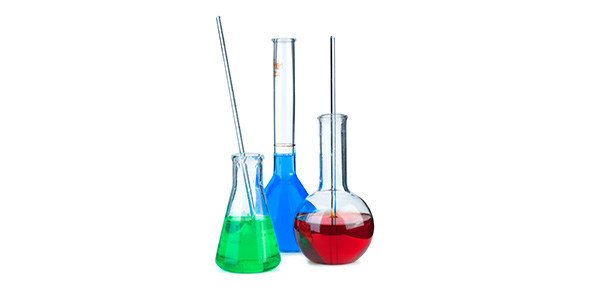# Chemical Interactions - Properties Of Carbon

13 Questions | Total Attempts: 177SettingsStudy of carbon and its properties

• 1.
What is the atomic number for carbon?
• A.

Six

• B.

Four

• C.

Two

• 2.
What is the hardest mineral formed by carbon?
• A.

Graphite

• B.

Carbonite

• C.

Diamond

• D.

Fullerenes

• E.

Nanotube

• 3.
In a nanotube, carbon atoms are arranged in a
• A.

Geodesic dome

• B.

Crystal structure

• C.

Ring

• D.

Long, hollow cylinder or tube

• 4.
What is the lead in a pencil?
• A.

Grapherene

• B.

Impure carbon

• C.

Graphite

• D.

Hydrocarbon

• 5.
How many valence electrons do carbon atoms have?
• A.

Two

• B.

Six

• C.

Four

• D.

Zero

• 6.
What are the ways that bonds between atoms can be represented?Make sure the answer includes all the ways.
• A.

Ring and branched

• B.

Branched, ring and straight

• C.

Straight and branched

• D.

Branched, ring, straight and tubed

• 7.
What is a chemical bond?
• A.

A force that holds branched bonds together

• B.

A force that holds chemical experiments together

• C.

A force that hods two carbons together

• D.

A force that holds two atoms together

• 8.
What is the melting point of diamond?
• A.

3000 degrees C

• B.

3500 degrees C

• C.

2500 degrees C

• D.

3500 degrees F

• 9.
Diamonds a re a sold substance that are
• A.

Hard and reactive

• B.

Hard and nonreactive

• C.

Slippery

• D.

Soft and nonreactive

• 10.
What is a nickname for fullerenes?
• A.

• B.

Buckydomes

• C.

Geodesic domes

• D.

Buckyballs

• 11.
What are nanotubes good conductors of?(Make sure the answer contains all possible conductors)
• A.

Electricity

• B.

Electricity and gas

• C.

Heat and electricity

• D.

Heat

• 12.
Why does graphite make an excellent lubricant?
• A.

Because it is so slippery

• B.

Because it is so hard

• C.

Because is is nonreactive

• D.

Because it is reactive

• 13.
Few elements have the ability of carbon to
• A.

Bond with itself in a couple of ways

• B.

Bond with itself and other elements in so many different ways

• C.

Bond with diamonds, graphites, fullerenes and nanotubes

• D.

Bond with only alkaline metals

Related TopicsBack to top